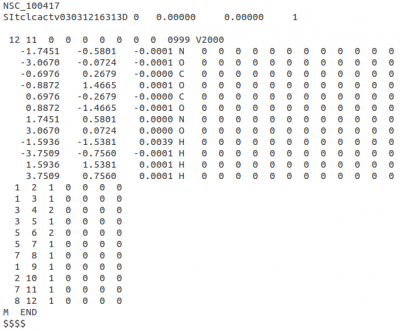# NEEMP:files

NEEMP uses 3 types of input files. SDF file contains structural information about the molecules, i.e. positions of atoms and bonding information; CHG file contains previously computed ab-initio charges; and PAR file stores the list of NEEMP's EEM parameters.

The following sections illustrate the details of each file type. Examples of these input files can also be found in the examples directory.

# SDF fileFigure 8: V2000 MOL record extracted from SDF file examples/set01.sdf. Note the atomic coordinates and bond connections blocks and the \$\$\$\$ line marking the end of the record.

SDF file contains MOL records for each molecule separated by line consisting only of four dollar signs, i.e. \$\$\$\$. Each MOL record can be either in V2000 or V3000 version. The latter one is required for molecules with more than 999 atoms or bonds. For further reference on MOL format, see specification: CTFile formats.

For convenience NEEMP has a support for reading SDF files which are compressed using gzip method. This might be useful when working with large databases of molecules.

# CHG file

Each record (charges for one molecule) in the CHG file consists of 3 parts. First line is the name of the molecule (it must be the same as in the SDF file to pair the charges to the structural information), second line contains number of atoms N and then N lines for each atom with its charges:

```My dummy molecule name
5
1  C   -0.921539
2  H   -0.507788
3  H   -0.565167
4  H   -0.200822
5  H    0.110252

```

Note that only the third column (the actual charge) is read, the first two are silently ignored. This implies that the order of the atoms in the structure and charge records must be the same to get a perfect pairing.

Every other record is separated from the previous one by a blank line.

# PAR file

PAR file is used for storing EEM parameters. It's a simple XML file of the following form. All fields are pretty self-explanatory. The AtomType parameter corresponds to the `--atom-types-by` option.

```<?xml version="1.0"?>
<ParameterSet>
<Parameters AtomType="ElemBond" Kappa="0.1976">
<Element Name=" O">
<Bond Type="1" A="2.6730" B="0.4091"/>
<Bond Type="2" A="2.7759" B="0.6434"/>
</Element>
<Element Name=" C">
<Bond Type="2" A="2.4880" B="0.2348"/>
<Bond Type="1" A="2.4787" B="0.2722"/>
</Element>
<Element Name=" H">
<Bond Type="1" A="2.3827" B="0.5701"/>
</Element>
<Element Name=" N">
<Bond Type="2" A="2.5440" B="0.2370"/>
<Bond Type="1" A="2.5370" B="0.2526"/>
</Element>
<Element Name=" S">
<Bond Type="2" A="2.4945" B="0.1454"/>
<Bond Type="1" A="2.4050" B="0.3687"/>
</Element>
</Parameters>
</ParameterSet>
```